4714.0 - National Aboriginal and Torres Strait Islander Social Survey, 2014-15
Latest ISSUE Released at 11:30 AM (CANBERRA TIME) 28/04/2016
 Page tools: .mffeedback,#pjs { display :none; } Enable Javascript to Print PagesPrint AllEmail NotificationRSSSearch this Product RELIABILITY OF ESTIMATES 1 The estimates in this publication are based on information obtained from a sample survey. Any data collection may encounter factors, known as non-sampling error, which can impact on the reliability of the resulting statistics. In addition, the reliability of estimates based on sample surveys are also subject to sampling variability. That is, the estimates may differ from those that would have been produced had all persons in the population been included in the survey. This is known as sampling error. Non-sampling error 2 Non-sampling error may occur in any collection, whether it is based on a sample or a full count such as a census. Sources of non-sampling error include non-response, errors in reporting by respondents or recording of answers by interviewers, and errors in coding and processing data. Every effort is made to reduce non-sampling error by careful design and testing of questionnaires, training and supervision of interviewers, and extensive editing and quality control procedures at all stages of data processing. Sampling error 3 One measure of the sampling error is given by the standard error (SE), which indicates the extent to which an estimate might have varied by chance because only a sample of persons was included. There are about two chances in three (67%) that a sample estimate will differ by less than one SE from the number that would have been obtained if all persons had been surveyed, and about 19 chances in 20 (95%) that the difference will be less than two SEs. 4 Another measure of the likely difference is the relative standard error (RSE), which is obtained by expressing the SE as a percentage of the estimate. The RSE is a useful measure in that it provides an immediate indication of the percentage errors likely to have occurred due to sampling, and thus avoids the need to refer also to the size of the estimate.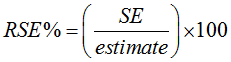5 The Excel spreadsheets in the Downloads tab contain all the tables produced for this release and the calculated RSEs for each of the estimates. 6 Only estimates (numbers or percentages) with RSEs less than 25% are considered sufficiently reliable for most analytical purposes. However, estimates with larger RSEs have been included. Estimates with an RSE in the range 25% to 50% should be used with caution while estimates with RSEs greater than 50% are considered too unreliable for general use. All cells in the Excel spreadsheets with RSEs greater than 25% contain a comment indicating the size of the RSE. These cells can be identified by a red indicator in the corner of the cell. The comment appears when the mouse pointer hovers over the cell. 7 The imprecision due to sampling variability, which is measured by the SE, should not be confused with inaccuracies that may occur because of imperfections in reporting by interviewers and respondents and errors made in coding and processing of data. Inaccuracies of this kind are referred to as the non-sampling error, and they may occur in any enumeration, whether it be in a full count or only a sample. In practice, the potential for non-sampling error adds to the uncertainty of the estimates caused by sampling variability. However, it is not possible to quantify the non-sampling error. 8 Another measure is the Margin of Error (MOE), which describes the distance from the population value that the sample estimate is likely to be within, and is specified at a given level of confidence. Confidence levels typically used are 90%, 95% and 99%. For example, at the 95% confidence level the MOE indicates that there are about 19 chances in 20 that the estimate will differ by less than the specified MOE from the population value (the figure obtained if all dwellings had been enumerated). The 95% MOE is calculated as 1.96 multiplied by the SE. 9 The 95% MOE can also be calculated from the RSE by: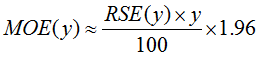10 The MOEs in this publication are calculated at the 95% confidence level. This can easily be converted to a 90% confidence level by multiplying the MOE by: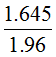or to a 99% confidence level by multiplying by a factor of: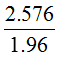11 A confidence interval expresses the sampling error as a range in which the population value is expected to lie at a given level of confidence. The confidence interval can easily be constructed from the MOE of the same level of confidence by taking the estimate plus or minus the MOE of the estimate. Standard errors of proportions and percentages 12 Proportions and percentages formed from the ratio of two estimates are also subject to sampling errors. The size of the error depends on the accuracy of both the numerator and the denominator. For proportions where the denominator is an estimate of the number of persons in a group and the numerator is the number of persons in a sub-group of the denominator group, the formula to approximate the RSE is given below. The formula is only valid when x is a subset of y.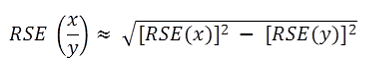13 For proportions where the denominator and numerator are independent estimates, for example a ratio of rates relating to two separate populations such as Aboriginal and Torres Strait Islander people and non-Indigenous people, the formula to approximate the RSE is given below. The formula is only valid when x and y are estimated from separate independent populations, and when the RSEs on x and y are small. Comparison of estimates 14 The difference between two survey estimates (counts or percentages) can also be calculated from published estimates. Such an estimate is also subject to sampling error. The sampling error of the difference between two estimates depends on their SEs and the relationship (correlation) between them. An approximate SE of the difference between two estimates (x-y) may be calculated by the following formula: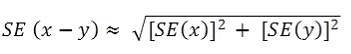15 While the above formula will be exact only for differences between separate sub-populations or uncorrelated characteristics of sub-populations, it is expected that it will provide a reasonable approximation for most differences likely to be of interest in this publication. Significance testing 16 For comparing estimates between surveys or between populations within a survey it is useful to determine whether apparent differences are 'real' differences between the corresponding population characteristics or simply the product of differences between the survey samples. One way to examine this is to determine whether the difference between the estimates is statistically significant. This is done by calculating the standard error of the difference between two estimates (x and y) and using that to calculate the test statistic using the formula below: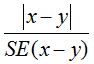where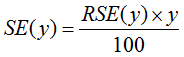17 If the value of the statistic is greater than 1.96 then we may say there is good evidence of a statistically significant difference at 95% confidence levels between the two populations with respect to that characteristic. Otherwise, it cannot be stated with confidence that there is a real difference between the populations. Age standardisation 18 Age standardisation techniques have been used in this publication to remove the effect of the differing age structures between the non-Indigenous and the Aboriginal and Torres Strait Islander populations. The age structure of the Aboriginal and Torres Strait Islander population is considerably younger than that of the non-Indigenous population, and age is strongly related to many health measures. Therefore, when making comparisons between the two populations, estimates of prevalence which do not take account of age may be misleading. The age standardised estimates of prevalence are those rates that 'would have occurred' should the Aboriginal and Torres Strait Islander population and the non-Indigenous population both have the standard age composition. 19 For this publication the direct age standardisation method was used. The standard population used was the total estimated resident population of Australia as at 30 June 2001. Estimates of age standardised rates were calculated using the following formula: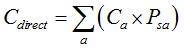20 Where Cdirect = the age standardised rate for the population of interest, a = the age categories that have been used in the age standardisation, Ca = the estimated rate for the population being standardised in age category a, and Psa = the proportion of the standard population in age category a. The age categories used in the standardisation for this publication are 15–24 years, 25–34 years, 35–44 years, 45–54 years and then 55 years and over. 21 Age standardisation may not be appropriate for particular variables, even though the populations to compare have different age distributions and the variables in question are related to age. It is also necessary to check that the relationship between the variable of interest and age is broadly consistent across the populations. If the rates vary differently with age in the two populations then there is evidence of an interaction between age and population, and as a consequence age standardised comparisons are not valid. Document Selection These documents will be presented in a new window.